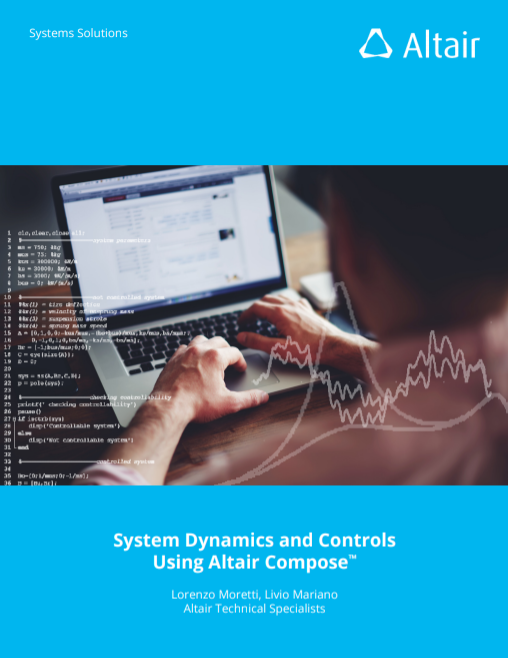This e-book is aimed at readers who are approaching “System Dynamics and Control Theory” for the first time or have a basic knowledge of this subject.  We will provide the reader all the required theoretical knowledge before moving to the practical examples shown.  If you are an expert in these fields, feel free to skip the theoretical explanation and focus on the practical examples solved using Altair Compose.

Fill out the form to download the e-book (as PDF file) + Compose scripts (as zipped .oml files).Contents:

1. Introduction
2. System Dynamics
1. Linear and Nonlinear Systems
2. Continuous and Discrete Dynamics
3. Time-variant and Time-invariant
4. SISO, SIMO, MIMO, MISO
3. Continuous Dynamics
1. System Analysis
2. Control Theory
4. Discrete Dynamics
1. Discretization and State-Space Representation
2. Natural and Forced Response
3. Eigenvalues and Eigenvectors
4. Stability
5. z Transform
6. Initial and Final Value Theorems
7. z Transfer Function
8. Discretization of Continuous Transfer Function
5. Appendix
1. Asymptotic Bode Plot
2. Proportional-Integral-Derivative (PID) GUI
3. Transformation from s-Domain to z-Domain
6. References